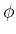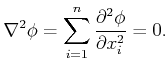### 8.4.4.4 Harmonic potential functions

Another important family of navigation functions is constructed from harmonic functions [236,239,240,481,529]. A functionis called a harmonic function if it satisfies the differential equation(8.49)

There are many possible solutions to the equation, depending on the conditions along the boundary of the domain over whichis defined. A simple disc-based example is given in  for which an analytical solution exists. Complicated navigation functions are generally defined by imposing constraints onalong the boundary of. A Dirichlet boundary condition means that the boundary must be held to a constant value. Using this condition, a harmonic navigation function can be developed that guides the state into a goal region from anywhere in a simply connected state space. If there are interior obstacles, then a Neumann boundary condition forces the velocity vectors to be tangent to the obstacle boundary. By solving (8.49) under a combination of both boundary conditions, a harmonic navigation function can be constructed that avoids obstacles by moving parallel to their boundaries and eventually landing in the goal. It has been shown under general conditions that navigation functions can be produced [240,239]; however, the main problems are that the boundary ofis usually not constructed explicitly (recall why this was avoided in Chapter 5) and that a numerical solution to (8.49) is expensive to compute. This can be achieved, for example, by using Gauss-Seidel iterations (as indicated in ), which are related to value iteration (see  for the distinction). A sampling-based approach to constructing navigation functions via harmonic functions is presented in . Value iteration will be used to produce approximate, optimal navigation functions in Section 8.5.2.

Steven M LaValle 2020-08-14